Like   Tweet   Pin   +1   in
 /* styles */ November 16th, 2017

Last week, MY Canada's 17th delegation took to Parliament Hill in the nation's capital to meet with Canadian MPs and Senators. Our mission was to thank and honour them for their hard work on behalf of Canadians, to share our hearts on a number of issues, and to ask them to work to advance godly legislation in the nation.

Some of the issues the team spoke to our leaders about this year include pornography, gender-selective abortion, informed consent for women considering abortion, the legalization of marijuana, government spending & national debt, freedom of conscience, religion and speech, and more.

In total, the team of 16 young adults had 57 sit-down meetings with Parliamentarians and hosted 25+ Members of Parliament plus staff at our parliamentary reception. It was a busy and impactful week!

## A Few Highlights:

The meetings themselves were fruitful as we were able to be a voice asking our leaders not to legalize marijuana and to safeguard the religious freedom of Canadians, among other issues. Incredibly, we also had opportunity to pray for most of the MPs and Senators with whom we met right in their parliamentary offices!

We sensed favour everywhere we went and seemed to have numerous unplanned encounters with leaders in hallways, elevators and sidewalks, including with leaders of the NDP and Green Party. A number of our last-minute meetings and reception guests were a direct result of these run-ins.

Our parliamentary reception was a huge success as we honoured our hard-working Members of Parliament and their incredible staff with a time of good food and entertainment. At this event, we specifically honoured MP Michelle Rempel for her tireless efforts to help persecuted Yazidi women and children, MP Ron McKinnon for his Good Samaritan Drug Overdose Act, and MP Garnett Genuis for his commitment to fighting for religious freedom in Canada and around the world.

Perhaps the biggest highlight for the team was being involved in the fight against Clause 14 of Bill C-51 which proposed removing specific protections for religious services and those performing them (Section 176) from the Criminal Code. We shared our concerns about this bill and its implications to the religious freedom of Canadians in every meeting for the first half of the week and were thrilled to be in the House of Commons celebrating the announcement that Section 176 would be safe on the Thursday of our delegation. What a victory! (Click here to read our National Update on this.)

We also saw tremendous growth in our delegates especially in their passion for Canada and being more involved in influencing the nation towards righteousness. A number of our young adults are prayerfully considering running in upcoming elections! We are so proud of this incredible group of Canadians.

## Thank you!

We simply could not have accomplished this mission with excellence without the support of the many people who partnered with us. From those who donated toward the delegation, to those who provided dinner meals for the team, to those who joined our intercessors e-mail list and the delegation team itself, this was a true group effort.

From the bottom of our hearts, we want to say "THANK YOU!" to everyone who sowed financially, prayed for and encouraged the team. Your prayers were needed and felt, and we could not have done it without you. Thank you for partnering with us. We did it, together!

## Next Steps:

Our 17th delegation was a huge success. But we know that this was just a starting point; we are believing that we will continue to see the impact from this mission and a real shift in our nation in the months to come. We are also beginning considerations for our next delegation! Will you help us keep the momentum going?

## Here's What You Can Do:

1) Pray: Please pray that the words we spoke in meetings and the prayers we prayed over MPs and Senators would take root and that hearts and minds would be impacted and leaders encouraged to stand for what is right. Please pray that our young adults would carry their new passion for Canada home to their families and churches and that the Church in Canada would become more involved in our government and democracy.

2) Donate: We are already budgeting for our next delegation. If you are able to help us get a leg up so that the next team is even more impactful, please click here to make a donation now. God bless you!

3) Be a Voice: Going to Parliament to meet with Canada's leaders is a wonderful example of our democracy. But you can be a powerful voice for righteousness right from where you are at. Please continue to read our National Updates and most importantly, take action, in the months ahead. We believe that now, more than ever, the Church needs to get in the game to impact Canada for godly values. Thank you for tracking these issues with us.

## A Glimpse at Our Week in Parliament:

Here is a sneak peak into our busy week on Parliament Hill from the many meetings to the reception fun and group shots on the Hill. Enjoy!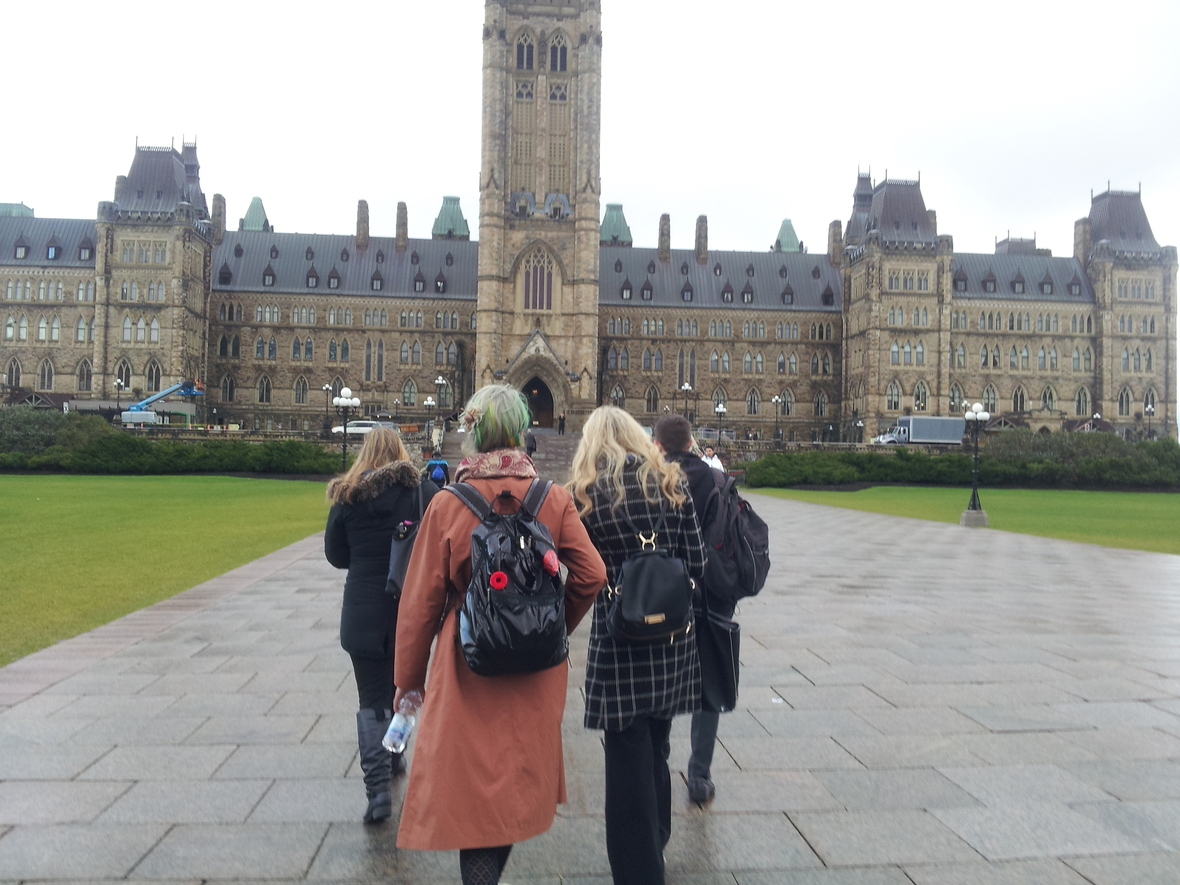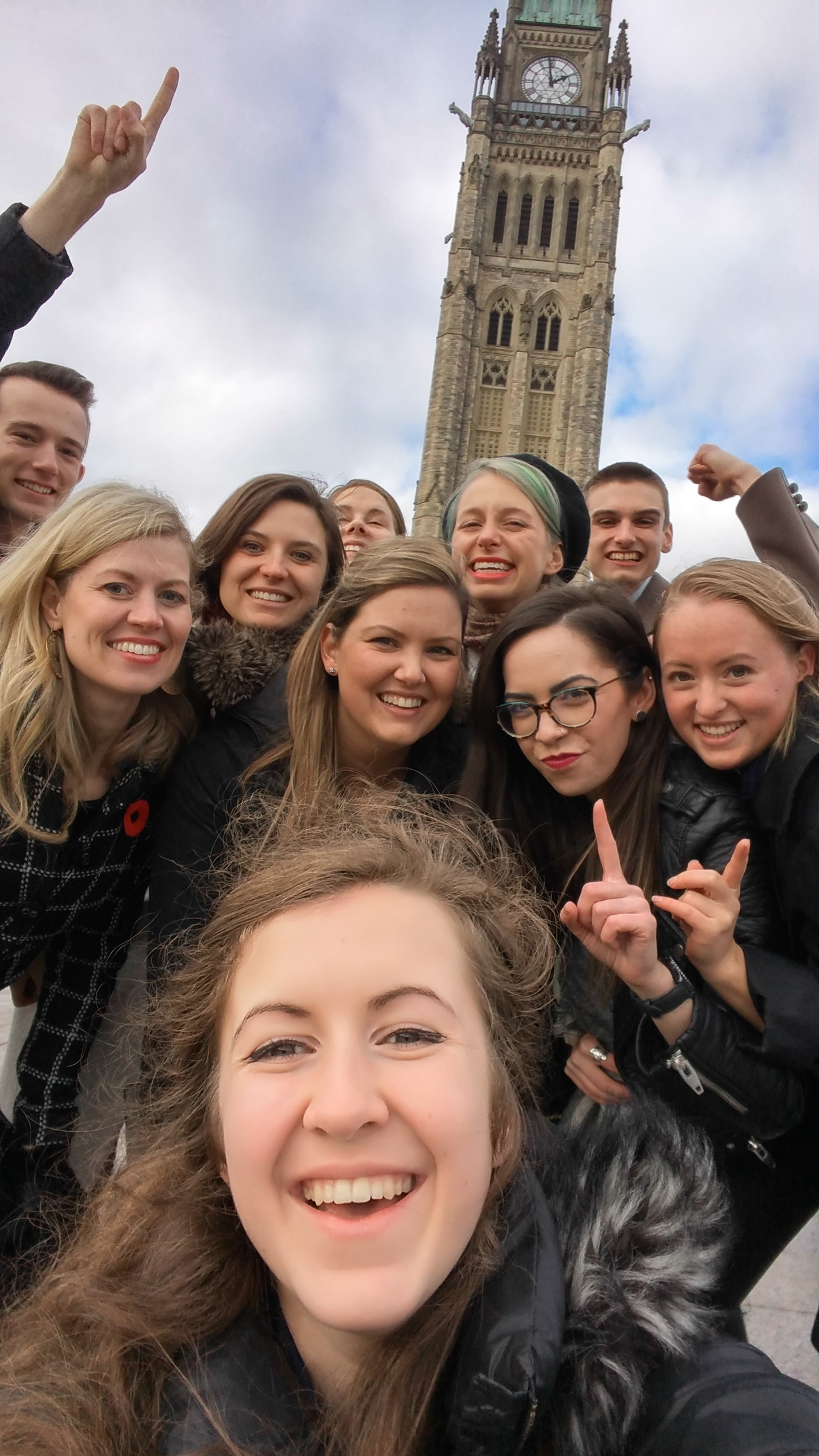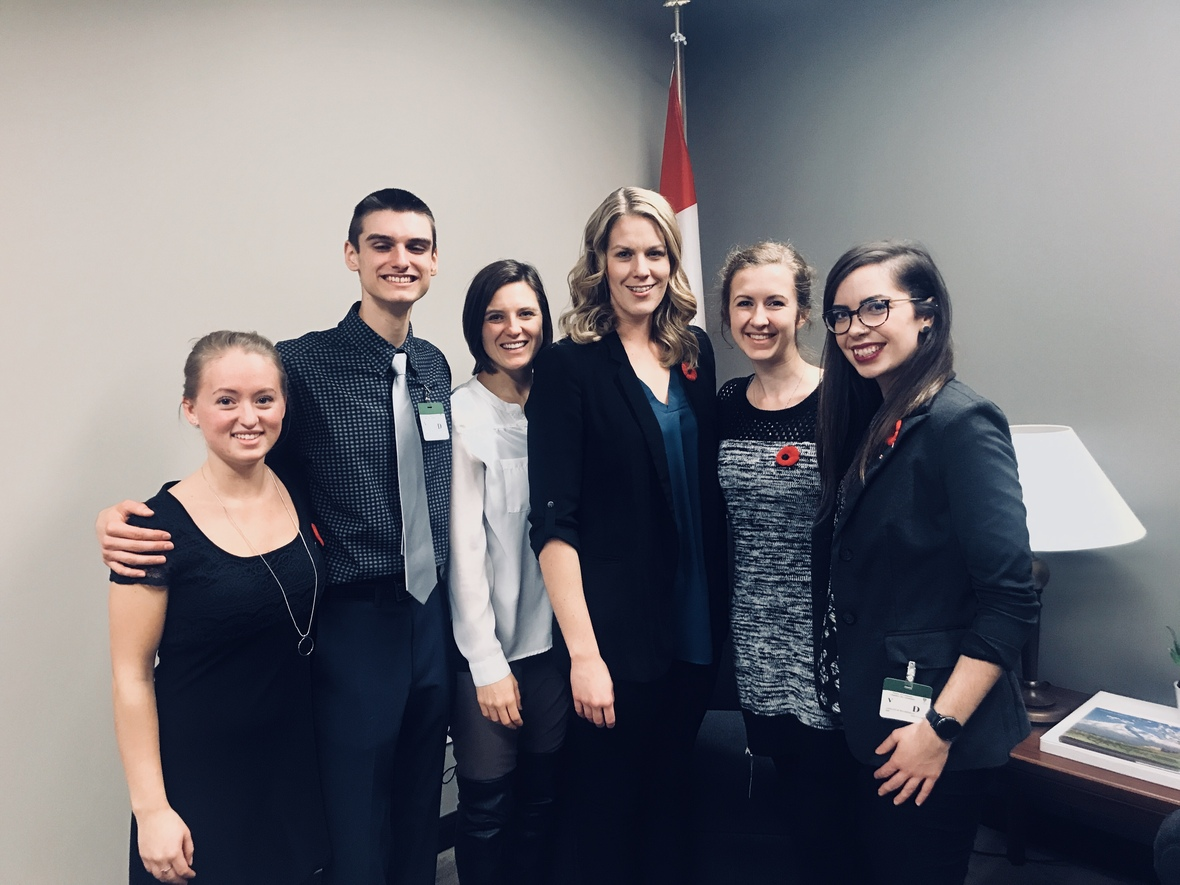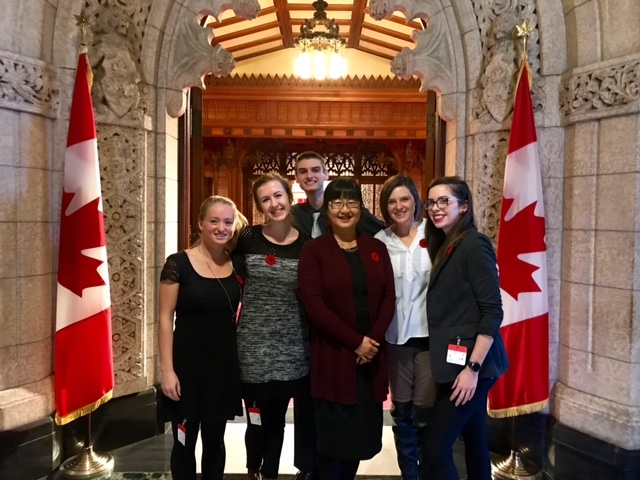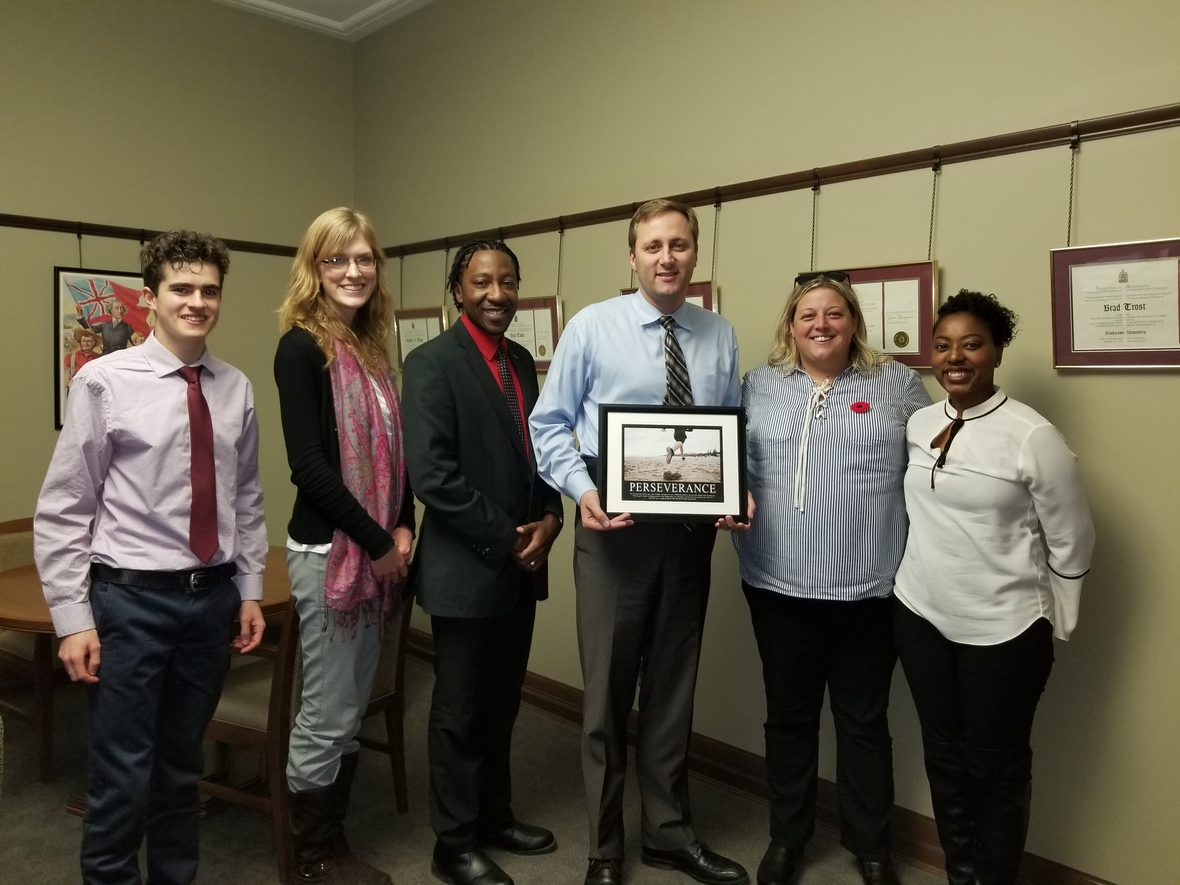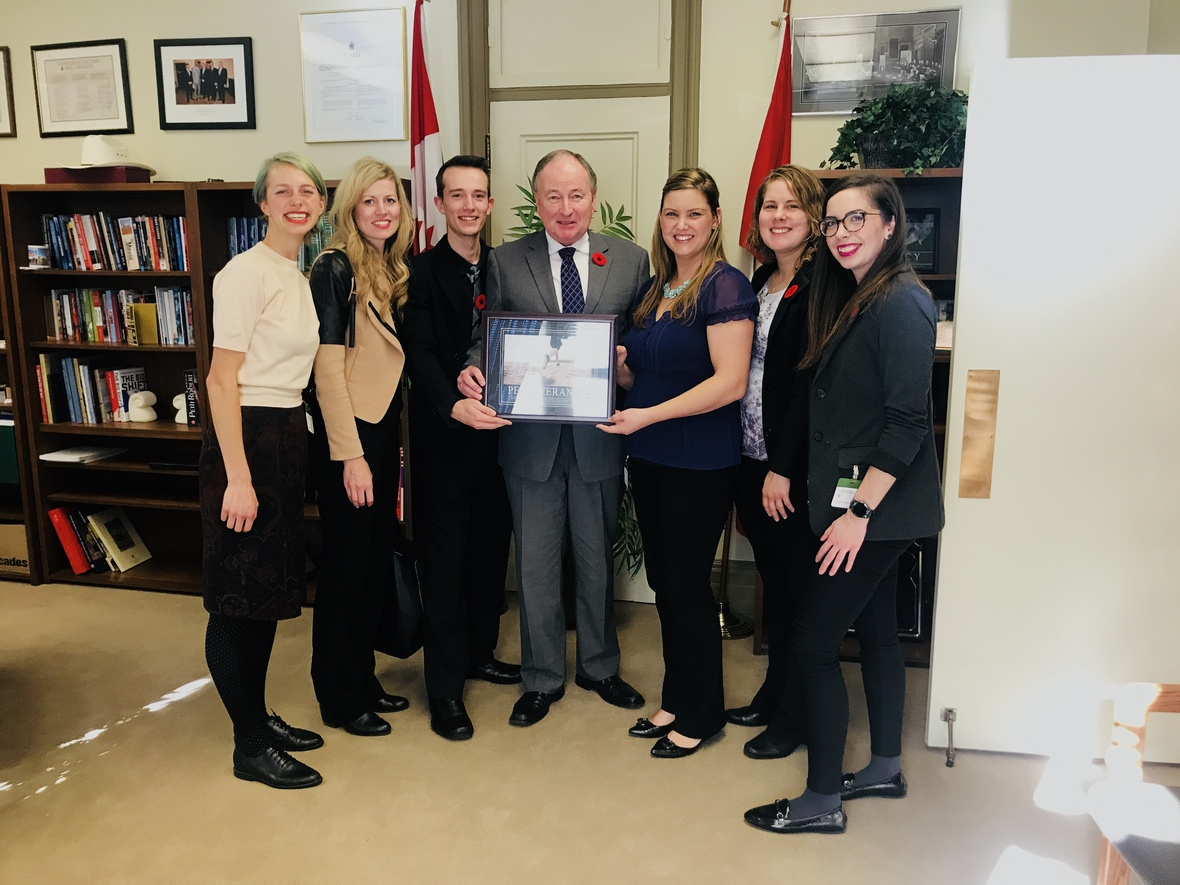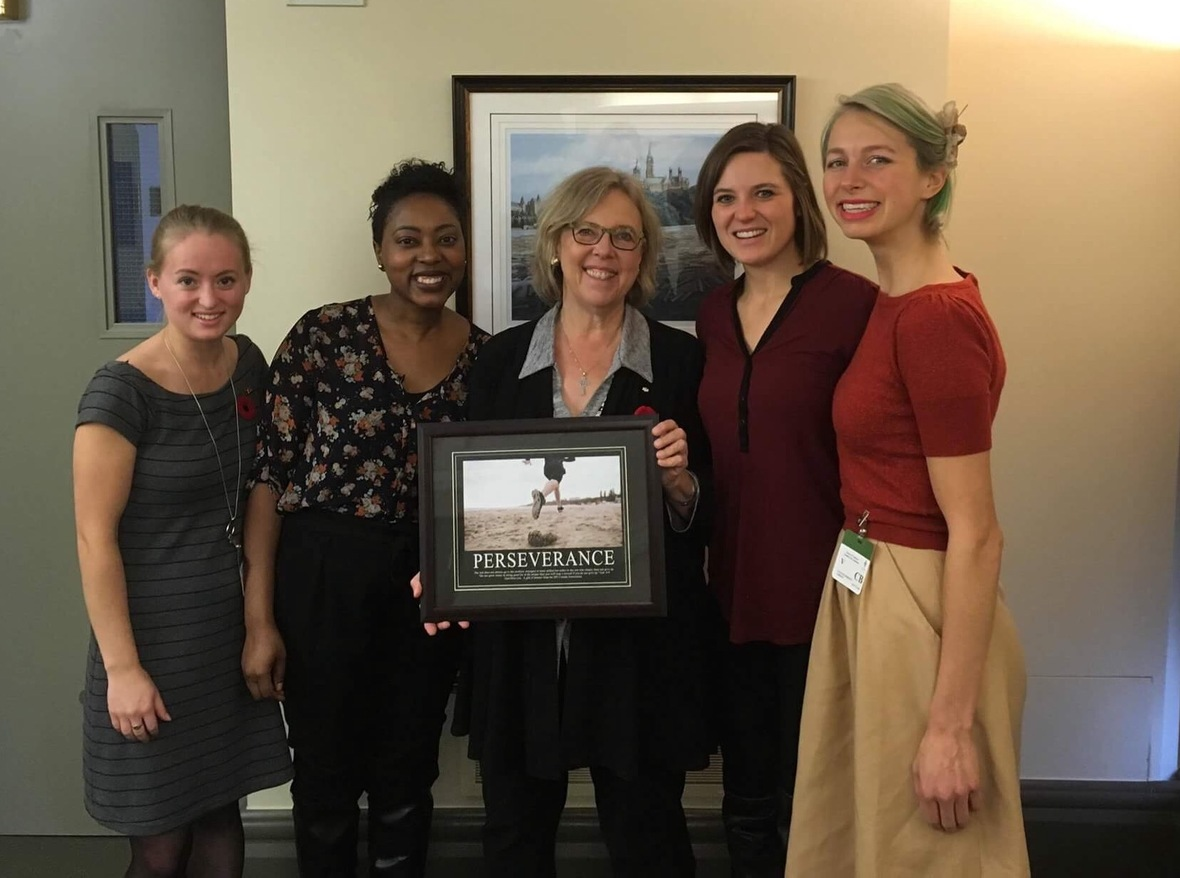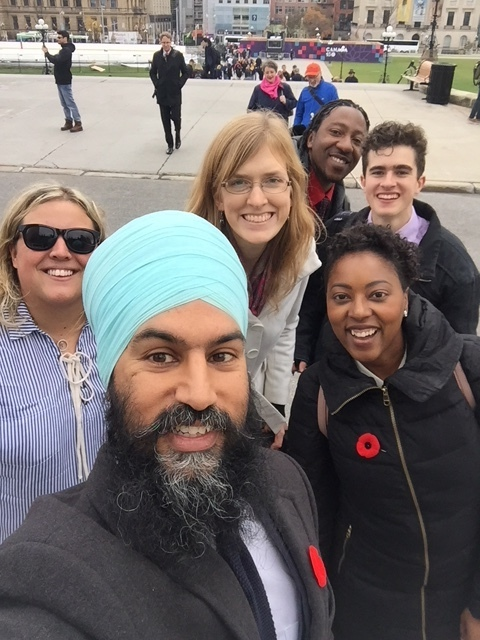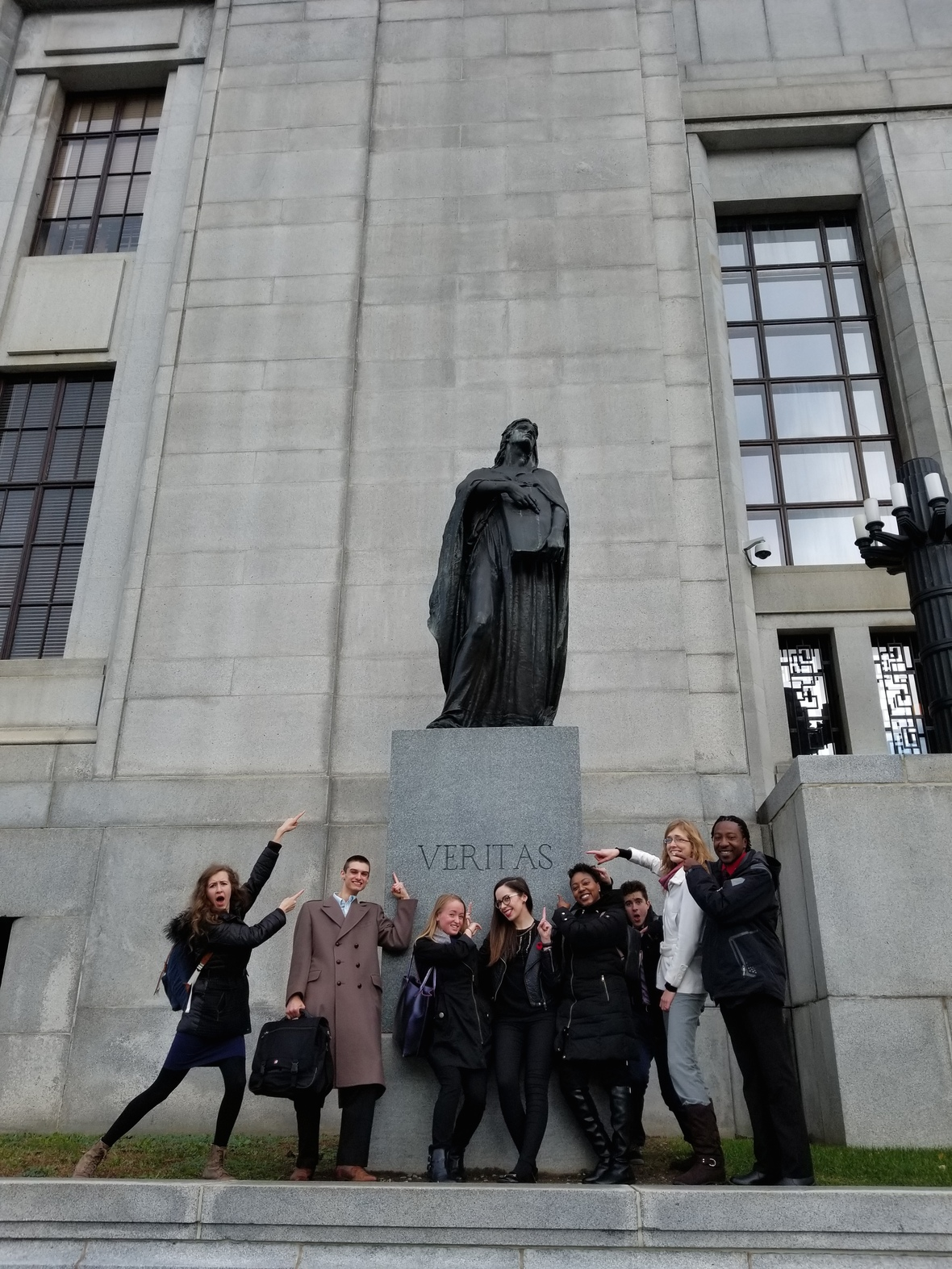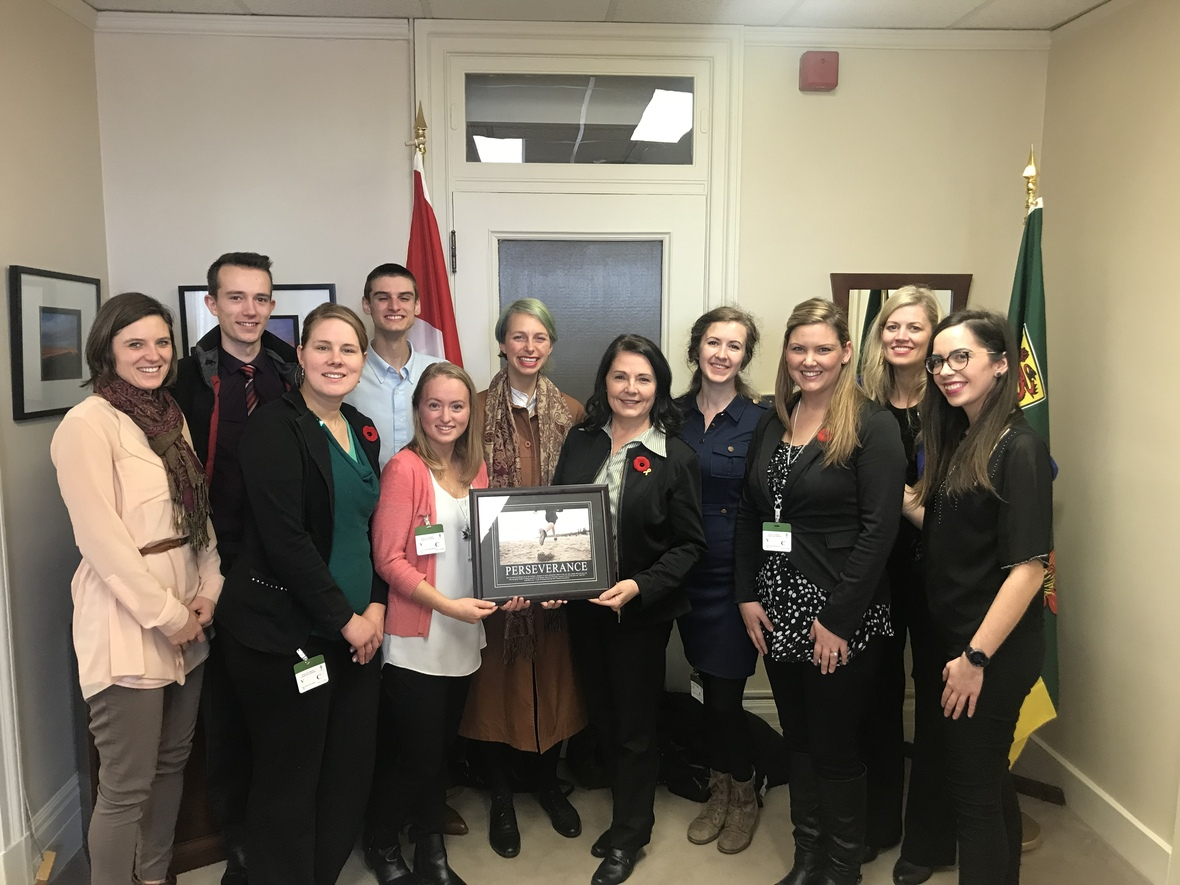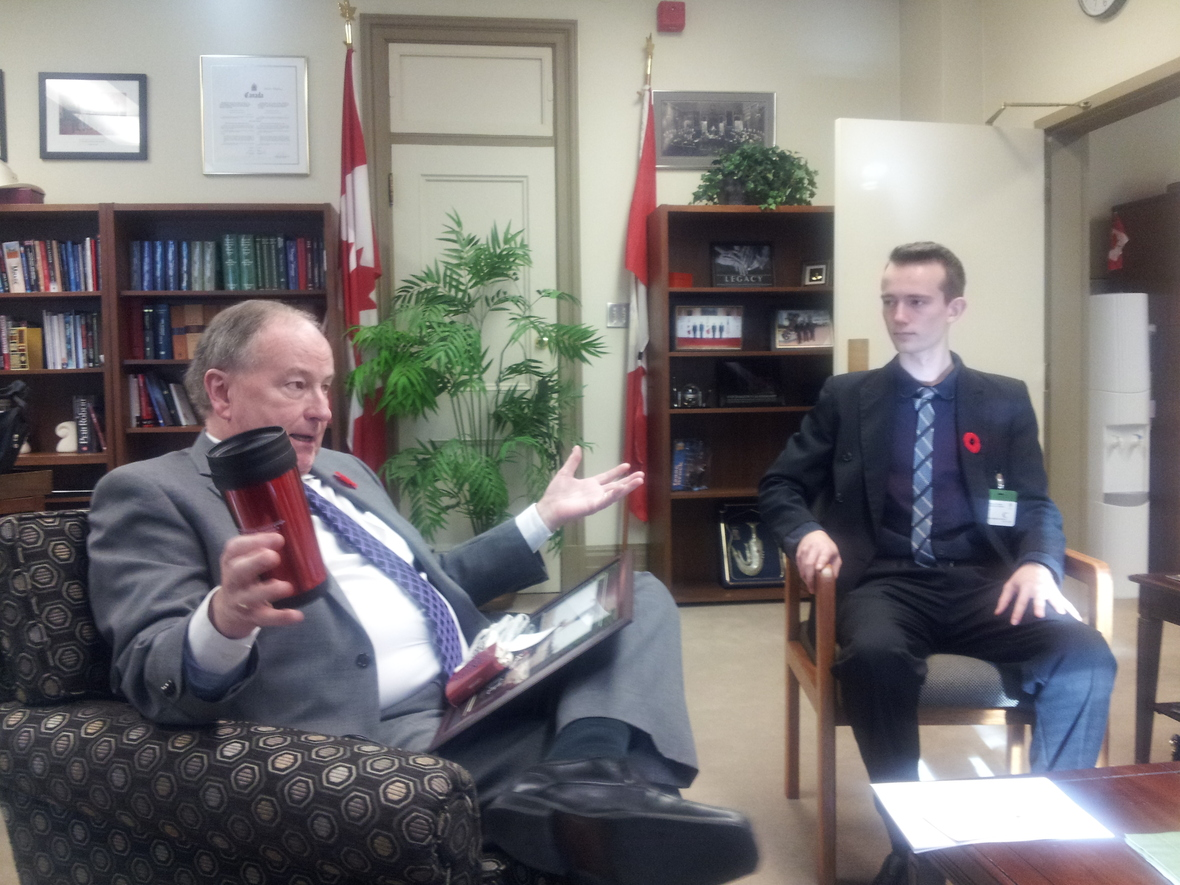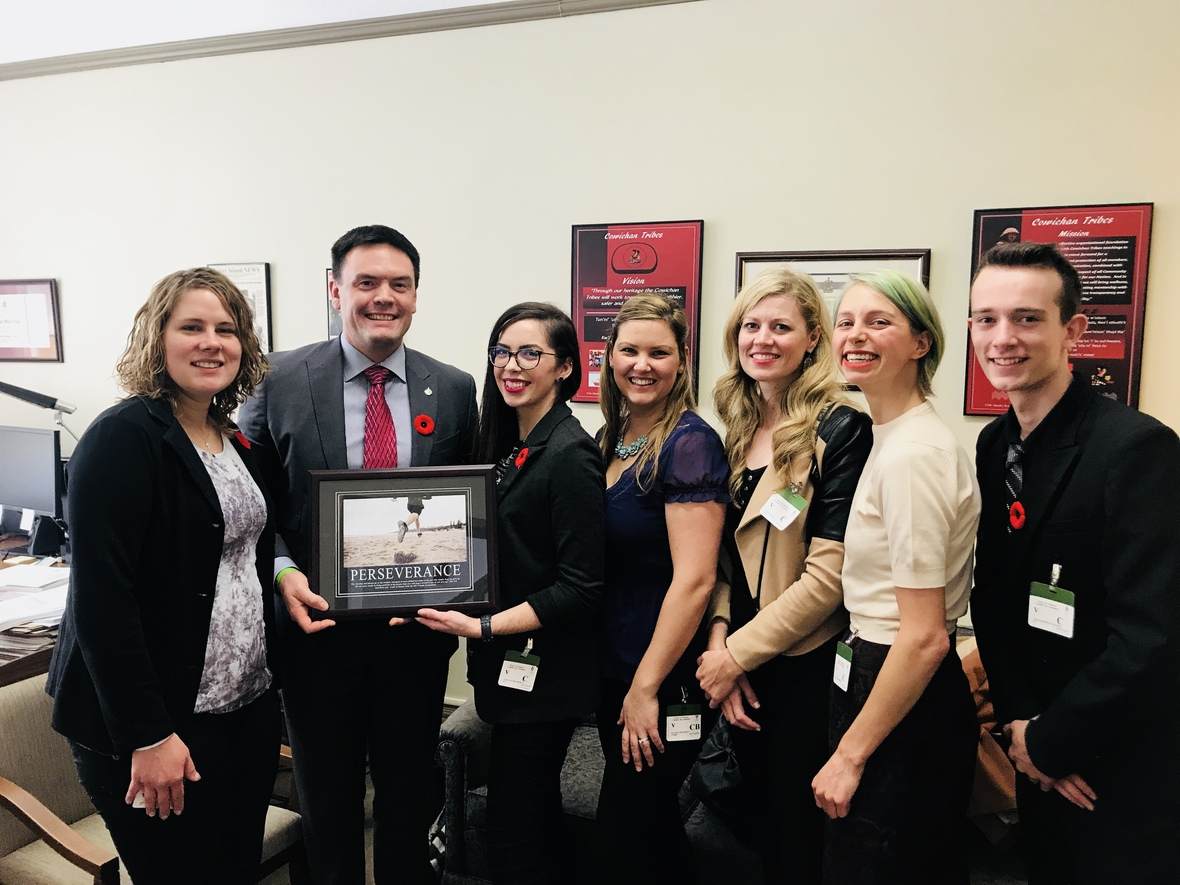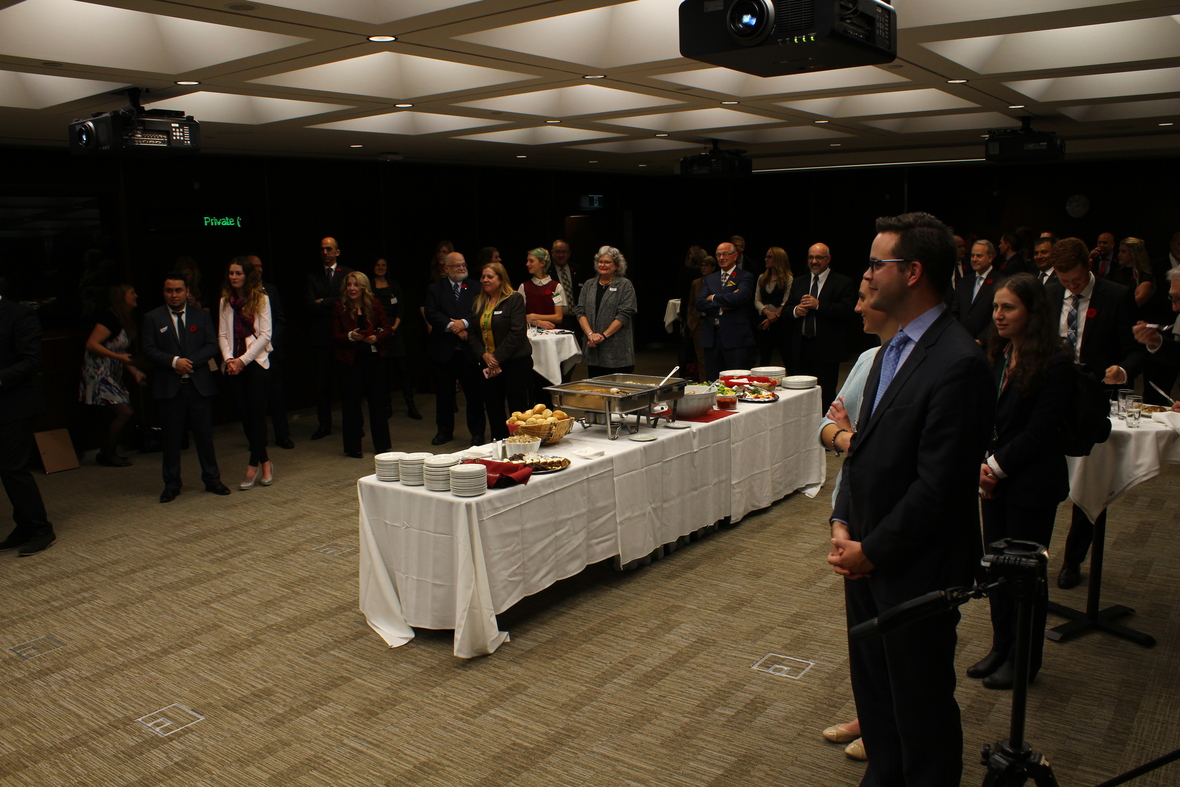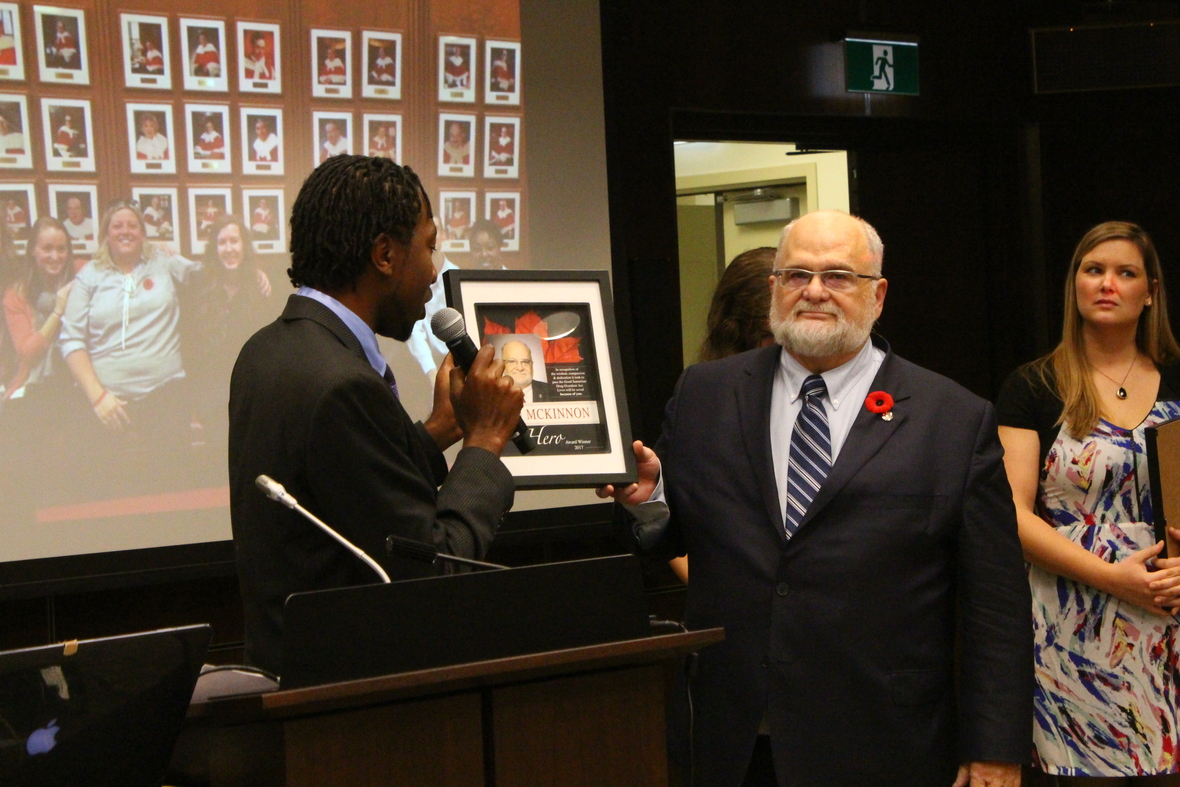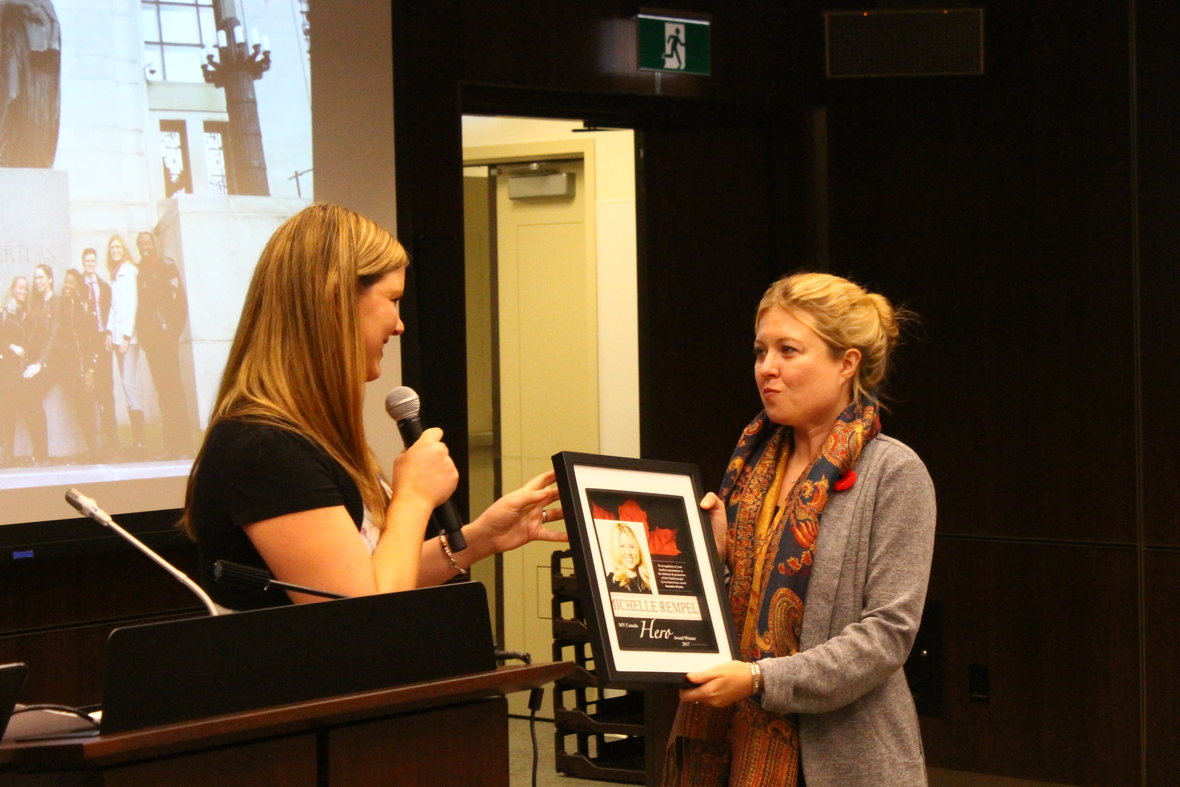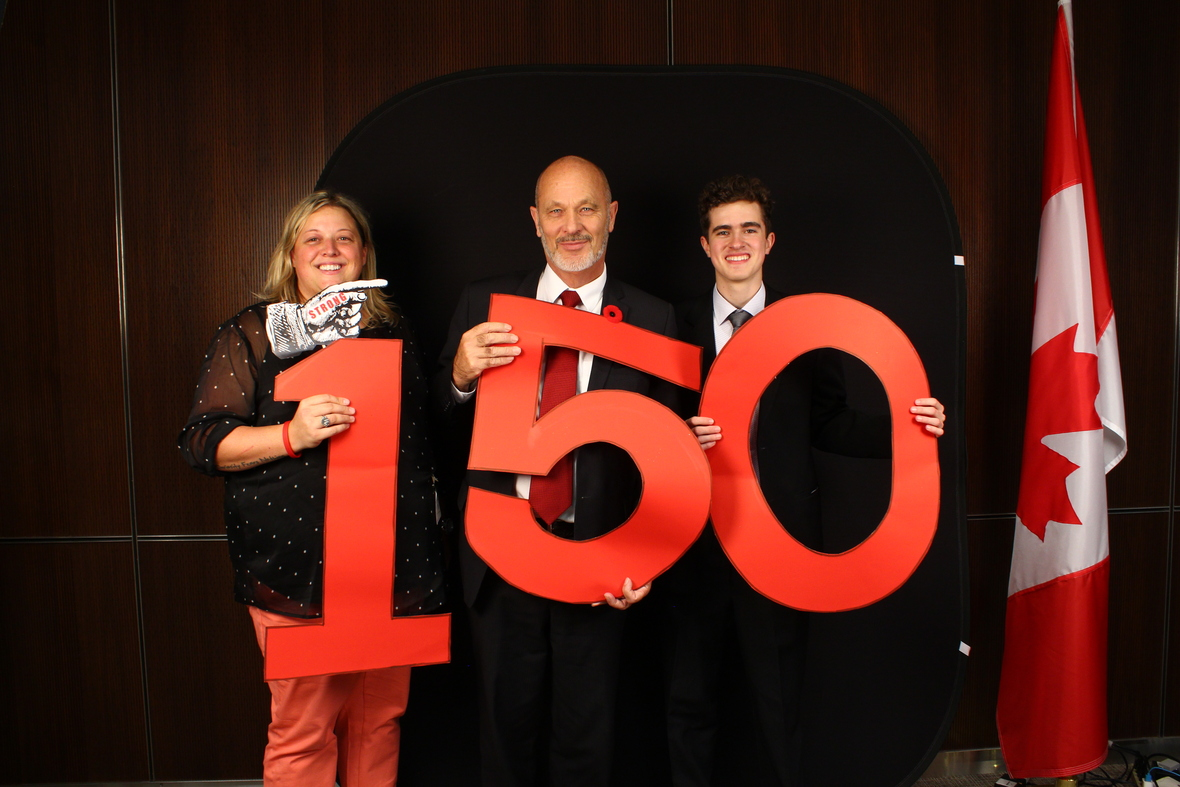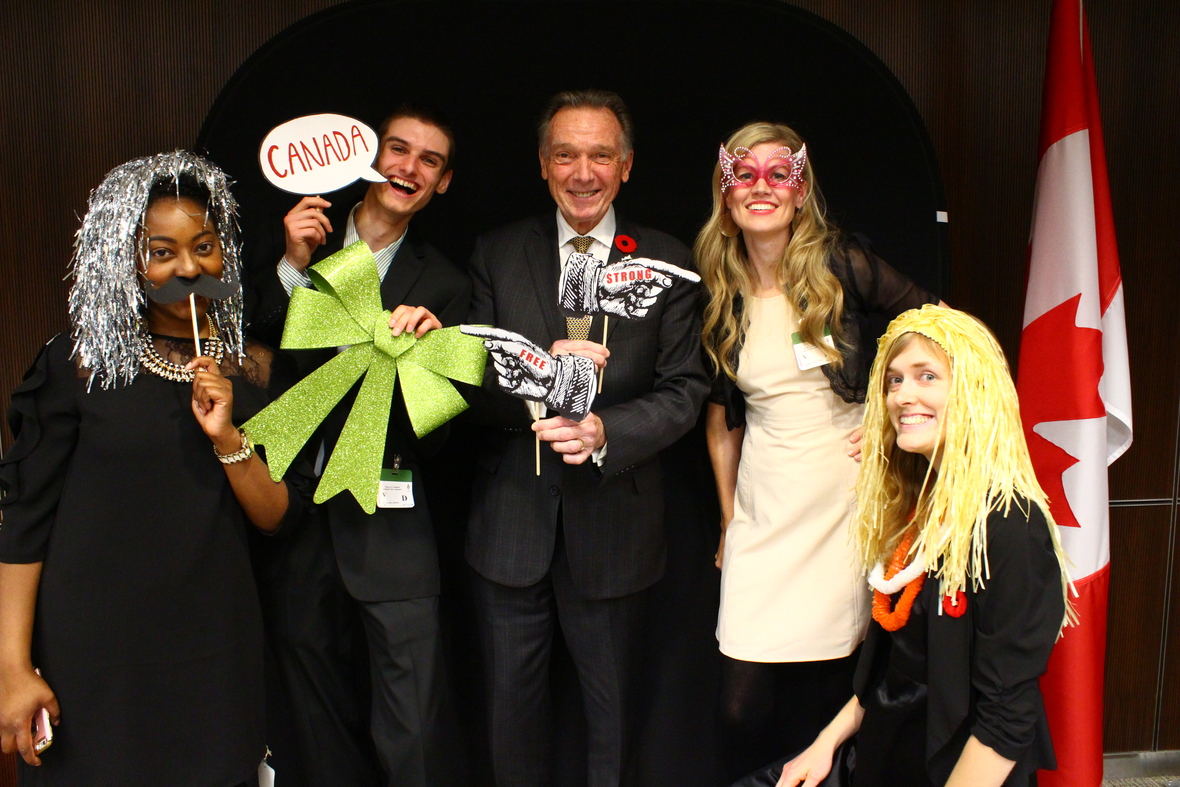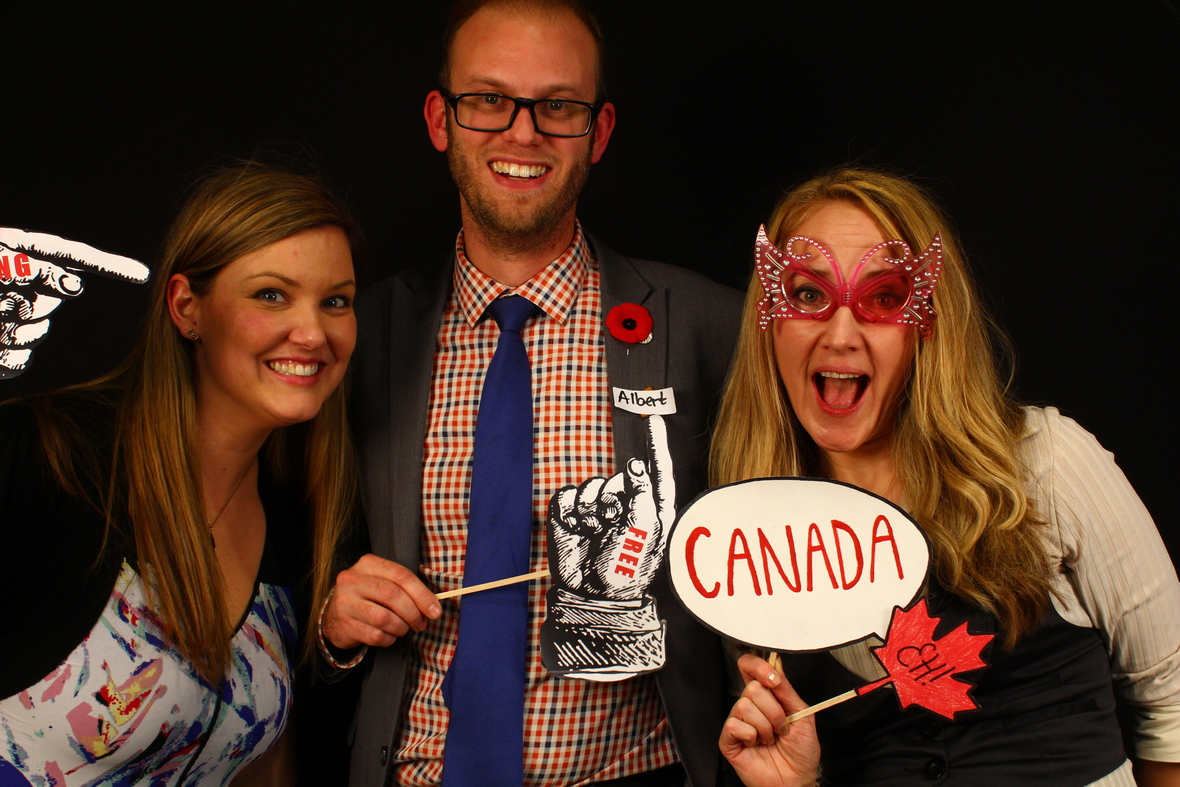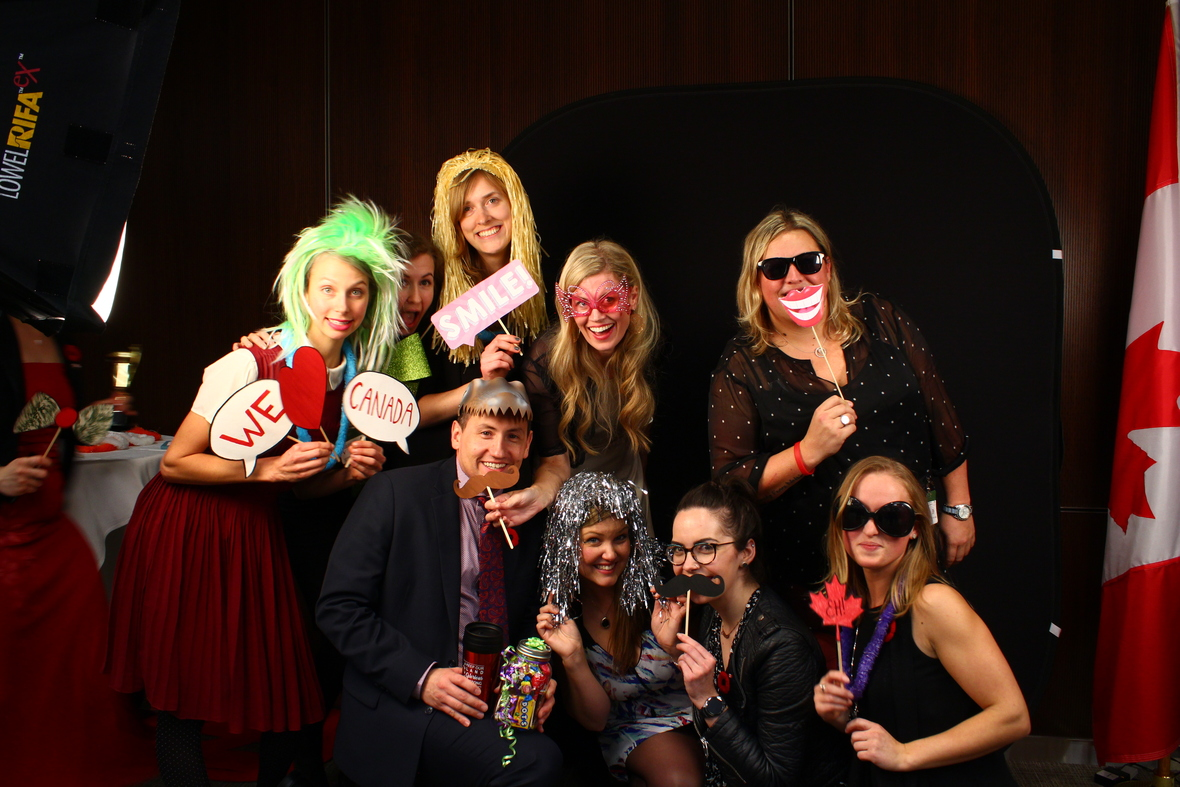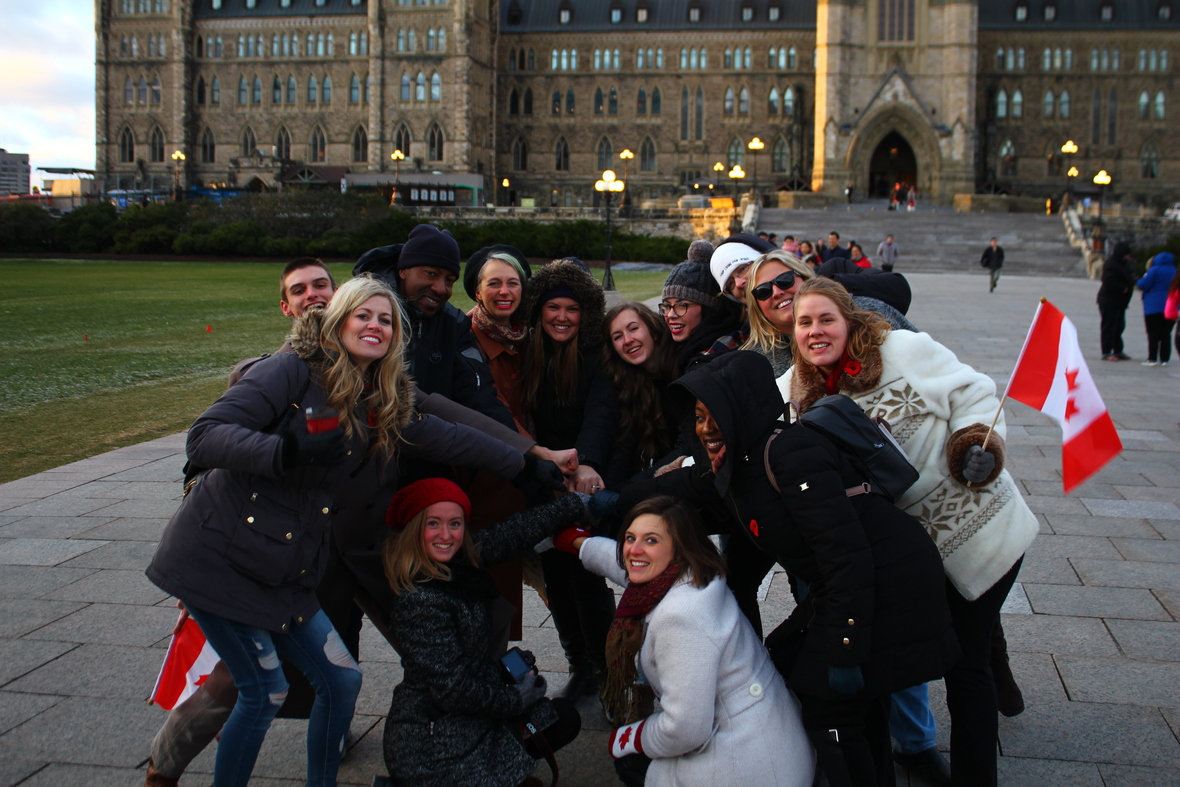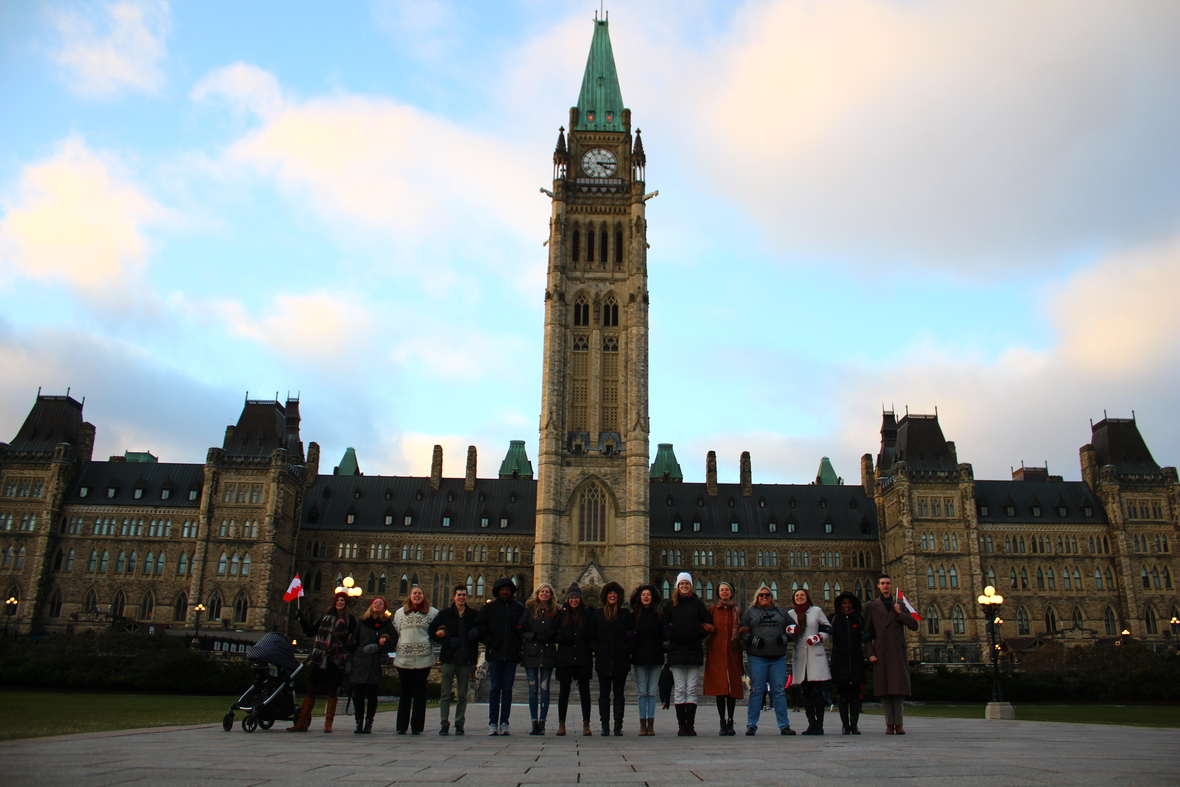table.module-19{width:98.49%;padding:0}table div table+table+table+table+table+table+table+table+table+table+table+table+table+table+table+table+table+table+table+table div table{width:98.49%;float:none;margin-left:auto;margin-right:auto;padding:0}table div table+table+table+table+table+table+table+table+table+table+table+table+table+table+table+table+table+table+table+table div table a{border:0 none;text-decoration:none}table div table+table+table+table+table+table+table+table+table+table+table+table+table+table+table+table+table+table+table+table div table img{width:100%!important;border:0 none;text-decoration:none}table div table+table+table+table+table+table+table+table+table+table+table+table+table+table+table+table+table+table+table+table div table td{width:100%;padding:0}/* styles */
 table div table+table+table+table+table+table+table+table+table+table+table+table+table+table+table+table+table+table+table+table+table div table{width:100%;padding:0}table div table+table+table+table+table+table+table+table+table+table+table+table+table+table+table+table+table+table+table+table+table div table img{width:96.23%;padding:0;float:none}table div table+table+table+table+table+table+table+table+table+table+table+table+table+table+table+table+table+table+table+table+table div table td{width:100%;padding:0 1.88% 18px}/* styles */## ANNOUNCEMENTS:

 table div table+table+table+table+table+table+table+table+table+table+table+table+table+table+table+table+table+table+table+table+table+table+table div table{width:100%;padding:0}table div table+table+table+table+table+table+table+table+table+table+table+table+table+table+table+table+table+table+table+table+table+table+table div table img{width:96.23%;padding:0;float:none}table div table+table+table+table+table+table+table+table+table+table+table+table+table+table+table+table+table+table+table+table+table+table+table div table td{width:100%;padding:0 1.88% 18px}/* styles */## Interested in Running for Election?

We can help! MY Canada is currently conducting a series of trainings and webinars to help godly candidates get started in their campaigns for office. Please e-mail admin@4mycanada.ca if you are interested in learning more.

 table div table+table+table+table+table+table+table+table+table+table+table+table+table+table+table+table+table+table+table+table+table+table+table+table+table div table{width:100%;padding:0}table div table+table+table+table+table+table+table+table+table+table+table+table+table+table+table+table+table+table+table+table+table+table+table+table+table div table img{width:96.23%;padding:0;float:none}table div table+table+table+table+table+table+table+table+table+table+table+table+table+table+table+table+table+table+table+table+table+table+table+table+table div table td{width:100%;padding:0 1.88% 18px}/* styles */## Volunteers Needed:

One of the most important things you can do to impact Canada is to volunteer to help Godly people get elected. Please e-mail us at admin@4mycanada.ca to sign up!

 table div table+table+table+table+table+table+table+table+table+table+table+table+table+table+table+table+table+table+table+table+table+table+table+table+table+table+table div table{width:100%;padding:0}table div table+table+table+table+table+table+table+table+table+table+table+table+table+table+table+table+table+table+table+table+table+table+table+table+table+table+table div table img{width:96.23%;padding:0;float:none}table div table+table+table+table+table+table+table+table+table+table+table+table+table+table+table+table+table+table+table+table+table+table+table+table+table+table+table div table td{width:100%;padding:0 1.88% 18px}/* styles */table div table+table+table+table+table+table+table+table+table+table+table+table+table+table+table+table+table+table+table+table+table+table+table+table+table+table+table+table div table td,table.module-27{width:100%;padding:0}table div table+table+table+table+table+table+table+table+table+table+table+table+table+table+table+table+table+table+table+table+table+table+table+table+table+table+table+table div table{width:100%;float:none;margin-left:auto;margin-right:auto;padding:0}table div table+table+table+table+table+table+table+table+table+table+table+table+table+table+table+table+table+table+table+table+table+table+table+table+table+table+table+table div table a{border:0 none;text-decoration:none}table div table+table+table+table+table+table+table+table+table+table+table+table+table+table+table+table+table+table+table+table+table+table+table+table+table+table+table+table div table img{width:100%!important;border:0 none;text-decoration:none}/* styles */
 table div table+table+table+table+table+table+table+table+table+table+table+table+table+table+table+table+table+table+table+table+table+table+table+table+table+table+table+table+table div table{width:100%;padding:0}table div table+table+table+table+table+table+table+table+table+table+table+table+table+table+table+table+table+table+table+table+table+table+table+table+table+table+table+table+table div table img{width:96.23%;padding:0;float:none}table div table+table+table+table+table+table+table+table+table+table+table+table+table+table+table+table+table+table+table+table+table+table+table+table+table+table+table+table+table div table td{width:100%;padding:0 1.88% 18px}/* styles */table div table+table+table+table+table+table+table+table+table+table+table+table+table+table+table+table+table+table+table+table+table+table+table+table+table+table+table+table+table+table div table,table.module-29{width:46.04%;float:left;padding:0}table div table+table+table+table+table+table+table+table+table+table+table+table+table+table+table+table+table+table+table+table+table+table+table+table+table+table+table+table+table+table div table a{border:0 none;text-decoration:none}table div table+table+table+table+table+table+table+table+table+table+table+table+table+table+table+table+table+table+table+table+table+table+table+table+table+table+table+table+table+table div table img{width:100%!important;border:0 none;text-decoration:none}table div table+table+table+table+table+table+table+table+table+table+table+table+table+table+table+table+table+table+table+table+table+table+table+table+table+table+table+table+table+table div table td{width:100%;padding:0 20px 20px 0}/* styles */ Thank you to everyone who came, prayed, served and sowed to make TheCRY Kingston a tremendous success! Videos from TheCRY Kingston are now available at www.thecrymovement.com
 table div table+table+table+table+table+table+table+table+table+table+table+table+table+table+table+table+table+table+table+table+table+table+table+table+table+table+table+table+table+table+table div table{width:100%;padding:0}table div table+table+table+table+table+table+table+table+table+table+table+table+table+table+table+table+table+table+table+table+table+table+table+table+table+table+table+table+table+table+table div table img{width:96.23%;padding:0;float:none}table div table+table+table+table+table+table+table+table+table+table+table+table+table+table+table+table+table+table+table+table+table+table+table+table+table+table+table+table+table+table+table div table td{width:100%;padding:0 1.88% 18px}/* styles */TheCRY Kingston special Canada 150 t-shirts are now on-sale while supplies last. Click here to order.

 table div table+table+table+table+table+table+table+table+table+table+table+table+table+table+table+table+table+table+table+table+table+table+table+table+table+table+table+table+table+table+table+table+table div table{width:100%;padding:0}table div table+table+table+table+table+table+table+table+table+table+table+table+table+table+table+table+table+table+table+table+table+table+table+table+table+table+table+table+table+table+table+table+table div table img{width:96.23%;padding:0;float:none}table div table+table+table+table+table+table+table+table+table+table+table+table+table+table+table+table+table+table+table+table+table+table+table+table+table+table+table+table+table+table+table+table+table div table td{width:100%;padding:0 1.88% 18px}/* styles */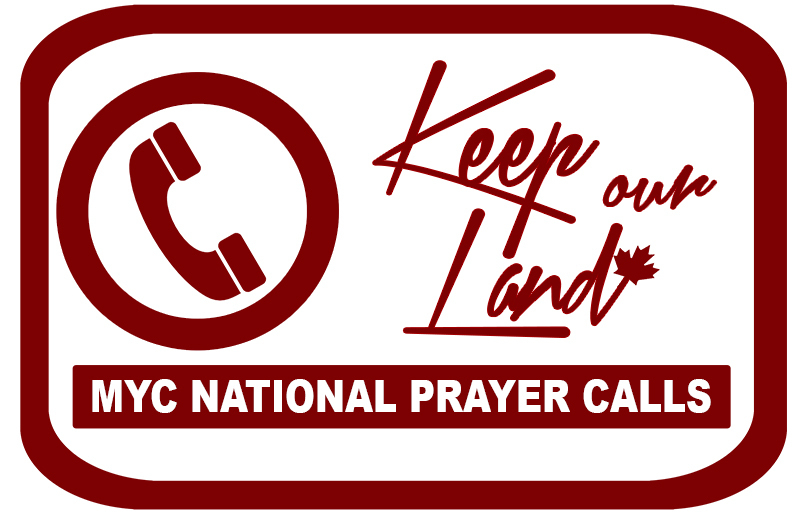MY Canada is hosting national prayer conference calls every second Tuesday at noon EST.

The next call is November 21st, 2017.

If you would like to participate in these calls please click here and add your name to the Keep Our Land e-list.

 table div table+table+table+table+table+table+table+table+table+table+table+table+table+table+table+table+table+table+table+table+table+table+table+table+table+table+table+table+table+table+table+table+table+table+table div table{width:100%;padding:0}table div table+table+table+table+table+table+table+table+table+table+table+table+table+table+table+table+table+table+table+table+table+table+table+table+table+table+table+table+table+table+table+table+table+table+table div table img{width:96.23%;padding:0;float:none}table div table+table+table+table+table+table+table+table+table+table+table+table+table+table+table+table+table+table+table+table+table+table+table+table+table+table+table+table+table+table+table+table+table+table+table div table td{width:100%;padding:0 1.88% 18px}/* styles */table div table+table+table+table+table+table+table+table+table+table+table+table+table+table+table+table+table+table+table+table+table+table+table+table+table+table+table+table+table+table+table+table+table+table+table+table+table div table{width:100%;padding:0}table div table+table+table+table+table+table+table+table+table+table+table+table+table+table+table+table+table+table+table+table+table+table+table+table+table+table+table+table+table+table+table+table+table+table+table+table+table div table img{width:96.23%;padding:0;float:none}table div table+table+table+table+table+table+table+table+table+table+table+table+table+table+table+table+table+table+table+table+table+table+table+table+table+table+table+table+table+table+table+table+table+table+table+table+table div table td{width:100%;padding:0 1.88% 18px}/* styles */